International
Tables for
Crystallography
Volume B
Reciprocal space
Edited by U. Shmueli

International Tables for Crystallography (2006). Vol. B, ch. 3.4, pp. 386-388   | 1 | 2 |

## Section 3.4.4. Preliminary derivation to obtain a formula which accelerates the convergence of an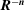sum over lattice points X(d)

D. E. Williamsa

aDepartment of Chemistry, University of Louisville, Louisville, Kentucky 40292, USA

### 3.4.4. Preliminary derivation to obtain a formula which accelerates the convergence of ansum over lattice points X(d)

| top | pdf |

The three-dimensional direct-space crystal lattice is specified by the origin vectors,and. A general vector in direct space is defined as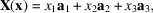where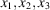are the fractional cell coordinates of X. A lattice vector in direct space is defined as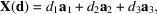where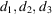are integers (specifying particular values of) designating a lattice point.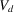is the direct-cell volume which is equal to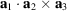. A general point in the direct lattice is X(x); the contents of the lattice are by definition identical as the components of x are increased or decreased by integer amounts.

The reciprocal-lattice vectors are defined by the relations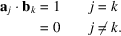A general vector in reciprocal space H(r) is defined as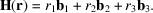A reciprocal-lattice vector H(h) is defined by the integer triplet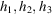(specifying particular values of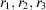) so that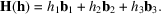In other sections of this volume a shortened notation h is used for the reciprocal-lattice vector. In this section the symbol H(h) is used to indicate that it is a particular value of H(r).

The three-dimensional Fourier transform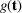of a function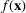is defined by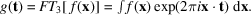The Fourier transform of the set of points defining the direct lattice is the set of points defining the reciprocal lattice, scaled by the direct-cell volume. It is useful for our purpose to express the lattice transform in terms of the Dirac delta function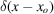which is defined so that for any function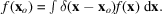We then write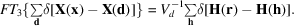First consider the lattice sum over the direct-lattice points X(d), relative to a particular point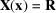, with omission of the origin lattice point.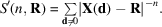The special case with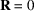will also be needed: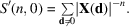Now define a sum of Dirac delta functions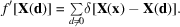Then S′ can be represented as an integral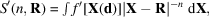in which a term is contributed to S′ whenever the direct-space vector X coincides with the lattice vector X(d), except for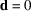. Now apply the convergence function to S′: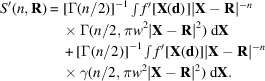The first integral is shown here only for the purpose of giving a consistent representation of S′; in fact, the first integral will be reconverted back into a sum and evaluated in direct space. The second integral will be transformed to reciprocal space using Parseval's theorem [see, for example, Arfken (1970)], which states that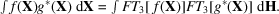Considering only the second integral in the formula for S′ and explicitly introducing theterm we have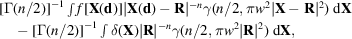where the unprimed f includes the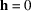term which was earlier omitted from f′: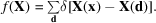Using Parseval's theorem, and evaluating the origin term, we have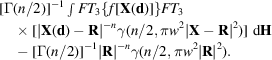The Fourier transform of the complement of the incomplete gamma function divided by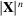is (Nijboer & DeWette, 1957)If there is a change of origin and the point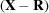is used instead of X the transform is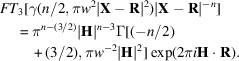Evaluation of the two Fourier transforms in the first term gives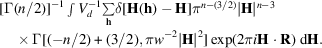Because of the presence of the Dirac delta function in each integral, we can convert the integrals with h unequal to zero into a sum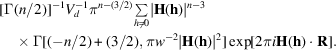Theterm needs to be evaluated in the limit. Clearly, the complex exponential goes to unity. If n is greater than 3 the limit of the indeterminate form infinity/infinity is needed: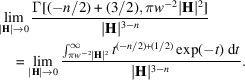The limit can be found by L'Hospital's rule [see, for example, Widder (1961)] which states that ifand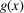both approach infinity as x approaches a constant, c, and the limit of the ratio of the first derivatives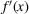and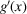exists, that limit is also true for the limit of the ratio of the functions: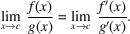To differentiate the definite integral function, Leibnitz's formula may be used [see, for example, Arfken (1970)]. This formula states that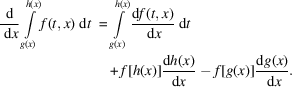In our case, x becomes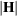; f becomes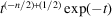which is independent of; g becomes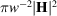; and h is infinite. Thus only the last term of Leibnitz's formula is nonzero and we obtain for the ratio of the first derivatives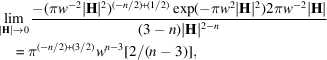so that the limiting value for theterm for n greater than 3 is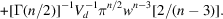The final result for S′ is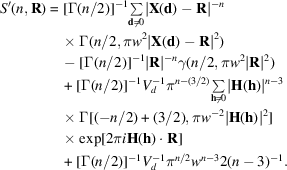The significance of the terms is as follows. The first term represents the convergence-accelerated direct sum, which does not include the origin term; the next term, also in direct space, corrects for the remainder resulting from the subtraction of the origin term; the third term comes from Parseval's theorem and is a sum over the nonzero h reciprocal-lattice points; and the last term is the reciprocal-latticeterm.

Ifthe second term becomes an indeterminate form 0/0. The limit can be found with use of L'Hospital's rule again, this time for the 0/0 form. We need the limit of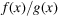, where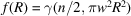and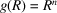. To differentiate the incomplete gamma function, we can again use Leibnitz's formula. In this case only the second term of the formula is nonzero and we obtain for the ratio of the first derivatives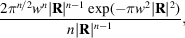so that the limiting value for this term as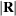approaches zero is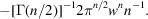Therefore, the value of the sum whenis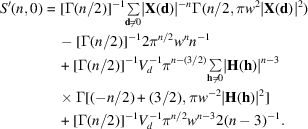### References

Arfken, G. (1970). Mathematical methods for physicists, 2nd ed. New York: Academic Press.
Nijboer, B. R. A. & DeWette, F. W. (1957). On the calculation of lattice sums. Physica (Utrecht), 23, 309–321.
Widder, D. V. (1961). Advanced calculus, 2nd ed. New York: Prentice-Hall.